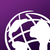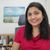# Extract average value at point.

956
2
01-11-2022 08:39 AMNew Contributor II

I have a dataset of over 500 points that represent the location of a number of site boundaries and a Tif file displaying the wind speed data over the study area. Is it possible using ArcGIS Pro to extract the wind speed data at these points?

Additionally would it be possible to take the mean wind speed within a certain distance of the point (or a polygon around a point).

Thanks,

Rob.

Tags (3)
1 Solution

Accepted SolutionsbyEsri Contributor
1. There are several tools available in the Spatial Analyst extension to perform this analysis.
• Extract Multi Values to Points tool will append your input points, and the extracted cell value will be added as a new field to the input points.
• Sample tool will create a table with the point location and the cell values.
• Extract Values to Points tool will create a new point feature with the extracted cell values.

2. From the problem you stated above, I am assuming you have one raster indicating wind speed (magnitude, not direction). The solution for wind direction will be different.

You can use the Buffer tool, and the Zonal Statistics tool or the Zonal Statistics as Table tool to solve this problem with wind speed.

First, create the buffer around the point. Use the Buffer tool to create a buffer with the desired distance around the point, and use the buffer output as zone, and wind speed as value raster in one of the following tools:

• Zonal Statistics tool will create a raster output, with the mean wind speed.
• Zonal Statistics as Table tool will create a table output, with the mean wind speed. Zonal Statistics as Table tool can also calculate statistics on overlapping buffer zones, if any, on ArcGIS Pro v2.5 or later.

Please note that the points and subsequently buffer polygons must have unique IDs, so that the mean is computed for each location.

Note: If you want to calculate the mean of wind direction, you should calculate Circular Mean, which will be available in a future release.

Hope this helps.
Thanks,
Sarmistha

2 RepliesNew Contributor

1. Extract Value to points

2. Calculate area if you want mean in area. if need a mean value at the points, try aggregate or block statistics first and then extract value to points.byEsri Contributor
1. There are several tools available in the Spatial Analyst extension to perform this analysis.
• Extract Multi Values to Points tool will append your input points, and the extracted cell value will be added as a new field to the input points.
• Sample tool will create a table with the point location and the cell values.
• Extract Values to Points tool will create a new point feature with the extracted cell values.

2. From the problem you stated above, I am assuming you have one raster indicating wind speed (magnitude, not direction). The solution for wind direction will be different.

You can use the Buffer tool, and the Zonal Statistics tool or the Zonal Statistics as Table tool to solve this problem with wind speed.

First, create the buffer around the point. Use the Buffer tool to create a buffer with the desired distance around the point, and use the buffer output as zone, and wind speed as value raster in one of the following tools:

• Zonal Statistics tool will create a raster output, with the mean wind speed.
• Zonal Statistics as Table tool will create a table output, with the mean wind speed. Zonal Statistics as Table tool can also calculate statistics on overlapping buffer zones, if any, on ArcGIS Pro v2.5 or later.

Please note that the points and subsequently buffer polygons must have unique IDs, so that the mean is computed for each location.

Note: If you want to calculate the mean of wind direction, you should calculate Circular Mean, which will be available in a future release.

Hope this helps.
Thanks,
Sarmistha